﻿ 改进的Winkler弹性地基模型在触地段动力分析中的应用
«上一篇文章快速检索 高级检索

 哈尔滨工程大学学报2018, Vol. 39Issue (9): 1451-1457  DOI: 10.11990/jheu.2017020500

### 引用本文DAI Yunyun, ZHOU Jing. Application of an improved Winkler elastic foundation-based model to the dynamic analysis of damaged pipelines in the touchdown zone[J]. Journal of Harbin Engineering University, 2018, 39(9), 1451-1457. DOI: 10.11990/jheu.201702050.### 文章历史

Application of an improved Winkler elastic foundation-based model to the dynamic analysis of damaged pipelines in the touchdown zone
DAI Yunyun, ZHOU JingState Key Laboratory of Coastal and Offshore Engineering, Dalian University of Technology, Dalian 116023, China
Abstract: To detect the dynamic response of a damaged pipeline in a riser located in a touchdown zone under complicated loads, an improved Winkler elastic foundation-based model is proposed to determine soil resistance and its distribution, taking into account the geological condition of the South China Sea. The finite element method was used to scatter the soil resistance into soil springs, and the correctness of the proposed model was verified by a large-scale indoor model test. The model was applied to the dynamic analysis of a full-sized damaged seabed pipeline, and the dynamic responses of the damaged pipeline in the touchdown zone were determined at different nodes. The results obtained were consistent with actual observations, and excellent application effects were achieved. The proposed model shows promising value for dealing with the practical problems associated with pipelines in touchdown zones.
Keywords: touchdown zone of riser    Winkler elastic foundation-based model    model test    volume damage    dynamic response    submarine pipeline

1 改进的Winkler弹性地基模型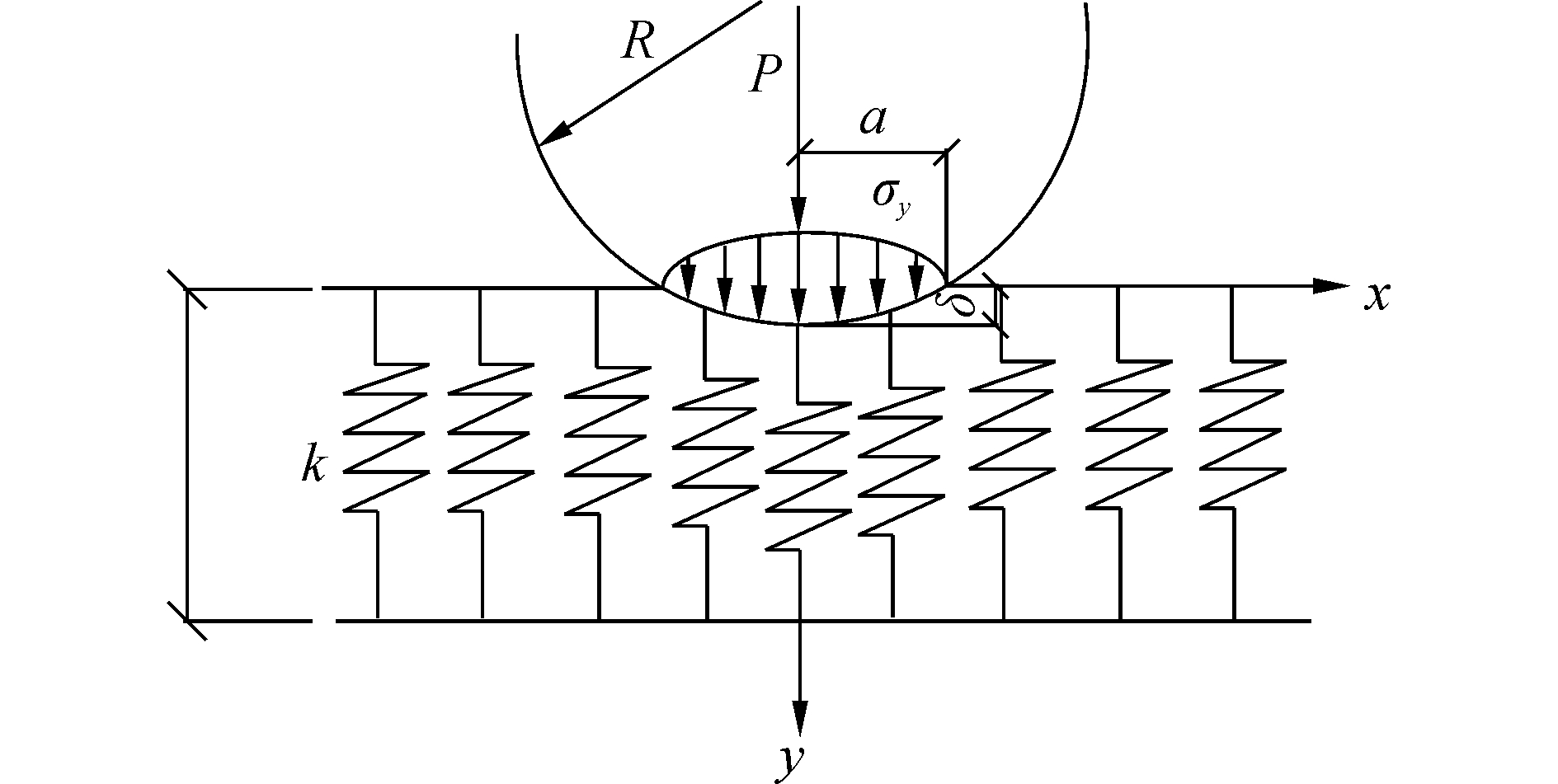Download: 图 1 管道压入Winkler弹性地基模型 Fig. 1 The model of pipeline on Winkler elastic foundation
1.1 理论推导

 $a = \sqrt {\frac{{4P{R^ * }}}{{{\rm{ \mathsf{ π} }}{E^ * }}}}$ (1)

 $\left\{ \begin{array}{l} {E^ * } = \left( {\frac{{1 - v_1^2}}{{{E_1}}} + \frac{{1 - v_2^2}}{{{E_2}}}} \right) - 1\\ {R^ * } = {\left( {\frac{1}{{{R_1}}} + \frac{1}{{{R_2}}}} \right)^{ - 1}} \end{array} \right.$ (2)

 $\left\{ \begin{array}{l} {E^ * } = {E_2}/\left( {1 - \nu _2^2} \right) = 1.10{E_2}\\ {R^ * } = {R_1} = R \end{array} \right.$ (3)

 $P = \frac{2}{3}\left( {\frac{{ka}}{h}} \right)\frac{{{a^2}}}{R}$ (4)
 $\delta = {a^2}/2R$ (5)

 ${\sigma _y}\left( x \right) = {\sigma _0}{\left[ {1 - {{\left( {\frac{x}{a}} \right)}^2}} \right]^{1/2}}$ (6)

 $k/h = 1.18{E^ * }/a$ (7)

 $P = \frac{2}{3}\left( {1.18{E^ * }} \right)\frac{{{a^2}}}{R}$ (8)

 ${a_{\max }} = D/3/2 = R/3$ (9)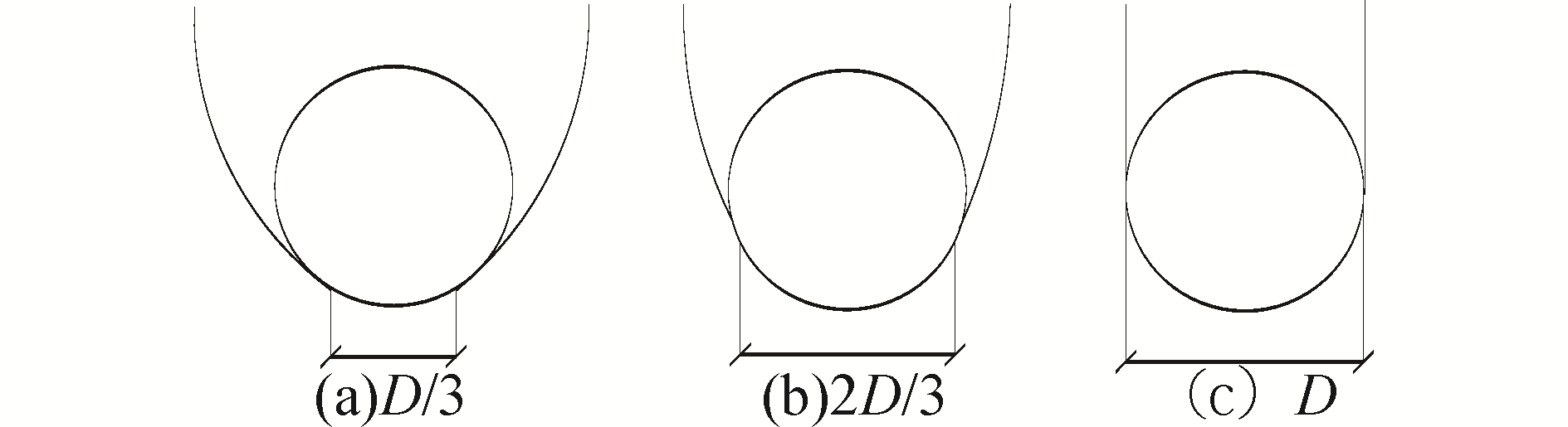Download: 图 2 不同的管土接触状态 Fig. 2 Pipe-soil contact status

a取最大值的时候，土体抗力达到最大。将式(9)代入式(8)，可得最大土体抗力PmaxR的关系为

 ${P_{\max }} = \frac{2}{3}\left( {1.18{E^ * }} \right)\frac{R}{9}$ (10)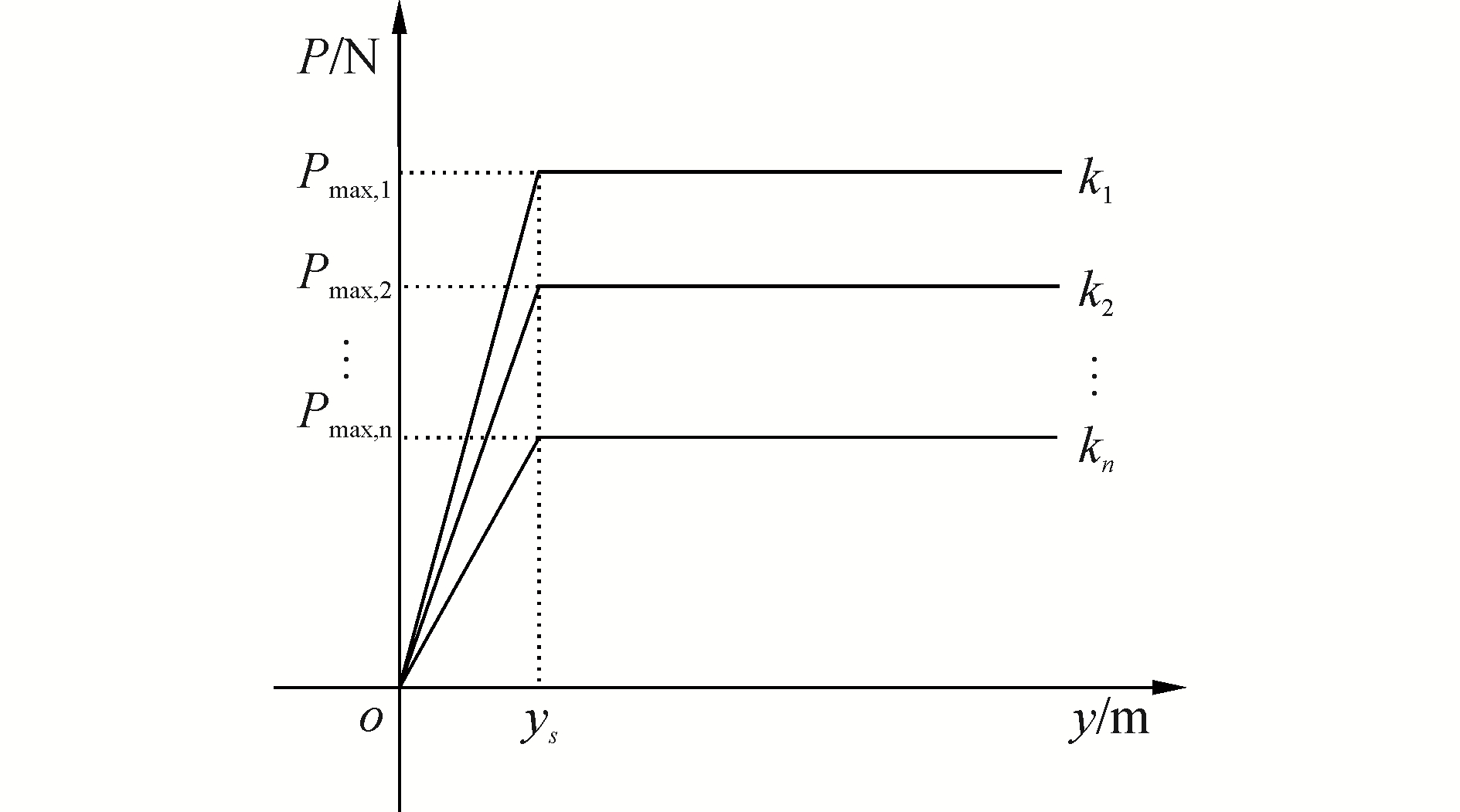Download: 图 3 等效海床竖向土弹簧的P-y曲线 Fig. 3 Equivalent P-y curves of seabed soil spring

 ${P_{\max ,n}} = \int_{{x_n} - 1}^{{x_n}} {{\sigma _y}\left( x \right){\rm{d}}x}$ (11)

1.2 建立改进的Winkler弹性地基模型

 ${k_n} = \frac{{{P_{\max ,n}}}}{{{y_s}}}$ (12)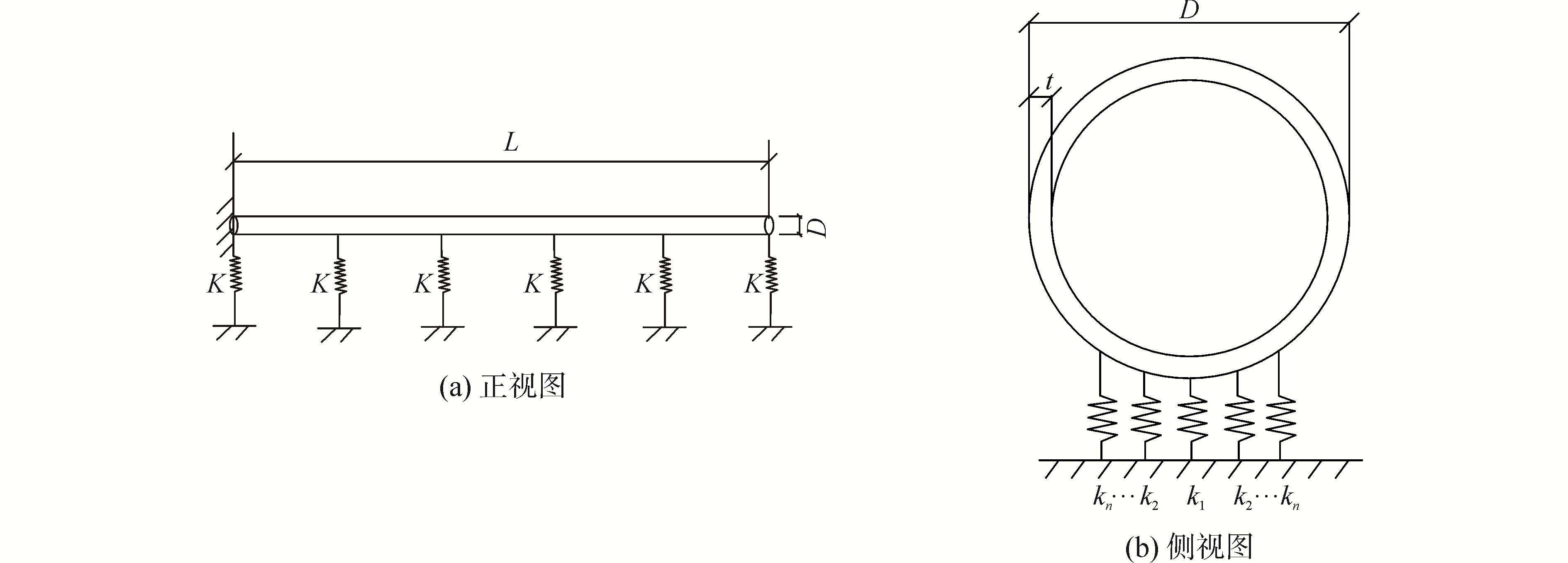Download: 图 4 改进的Winkler弹性地基模型改进的Winkler弹性地基模型 Fig. 4 Sketch of improved Winkler elastic foundation model

2 改进模型的试验验证 2.1 试验装置Download: 图 5 大尺寸管土相互作用的模型试验 Fig. 5 The large scale pipe-soil system
2.1.1 固结海床

2.1.2 管道模型

2.1.3 传感器的布置

2.2 模型验证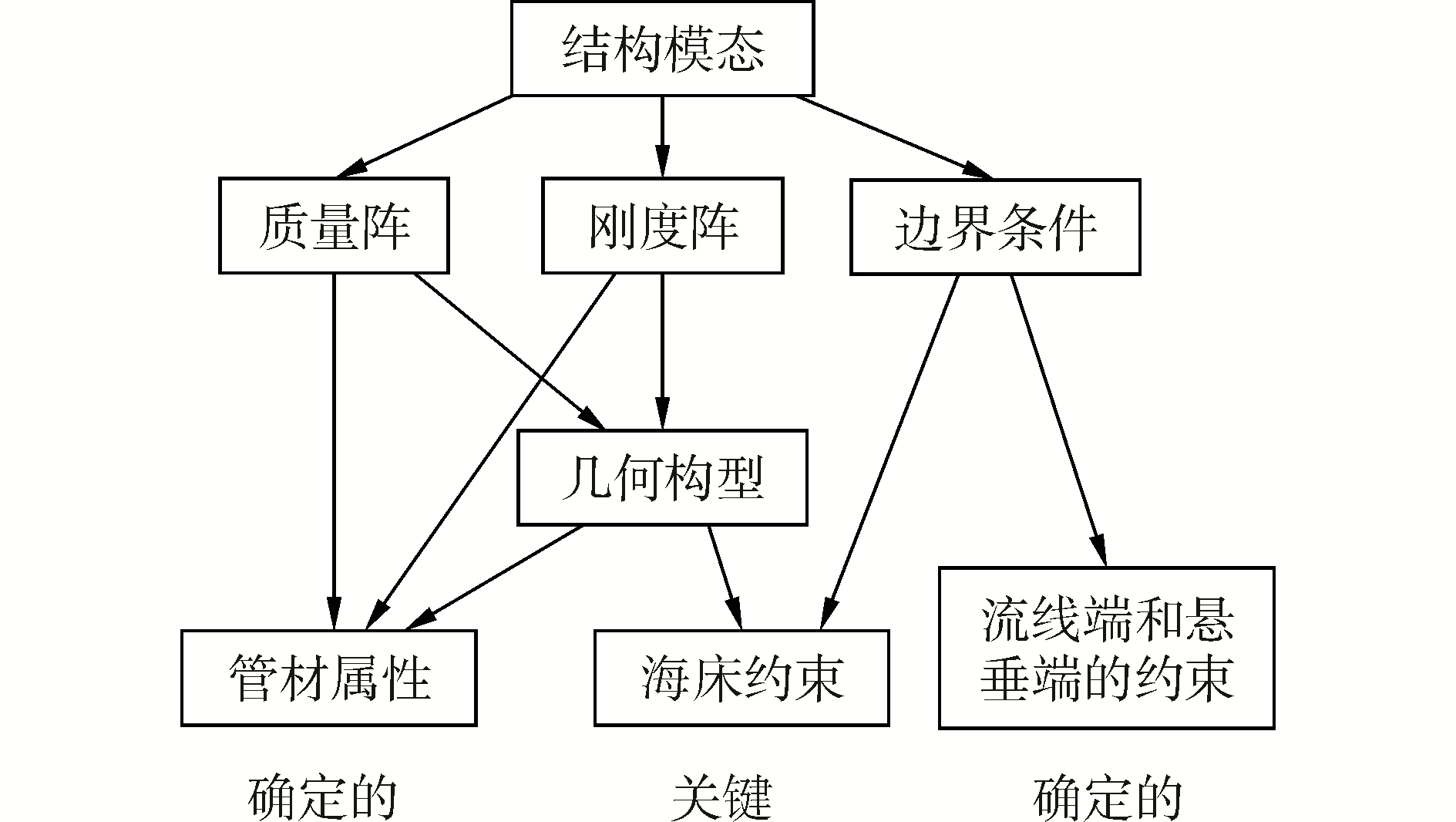Download: 图 6 模型的验证过程 Fig. 6 Validation procedure of the improved model
2.2.1 建立有限元模型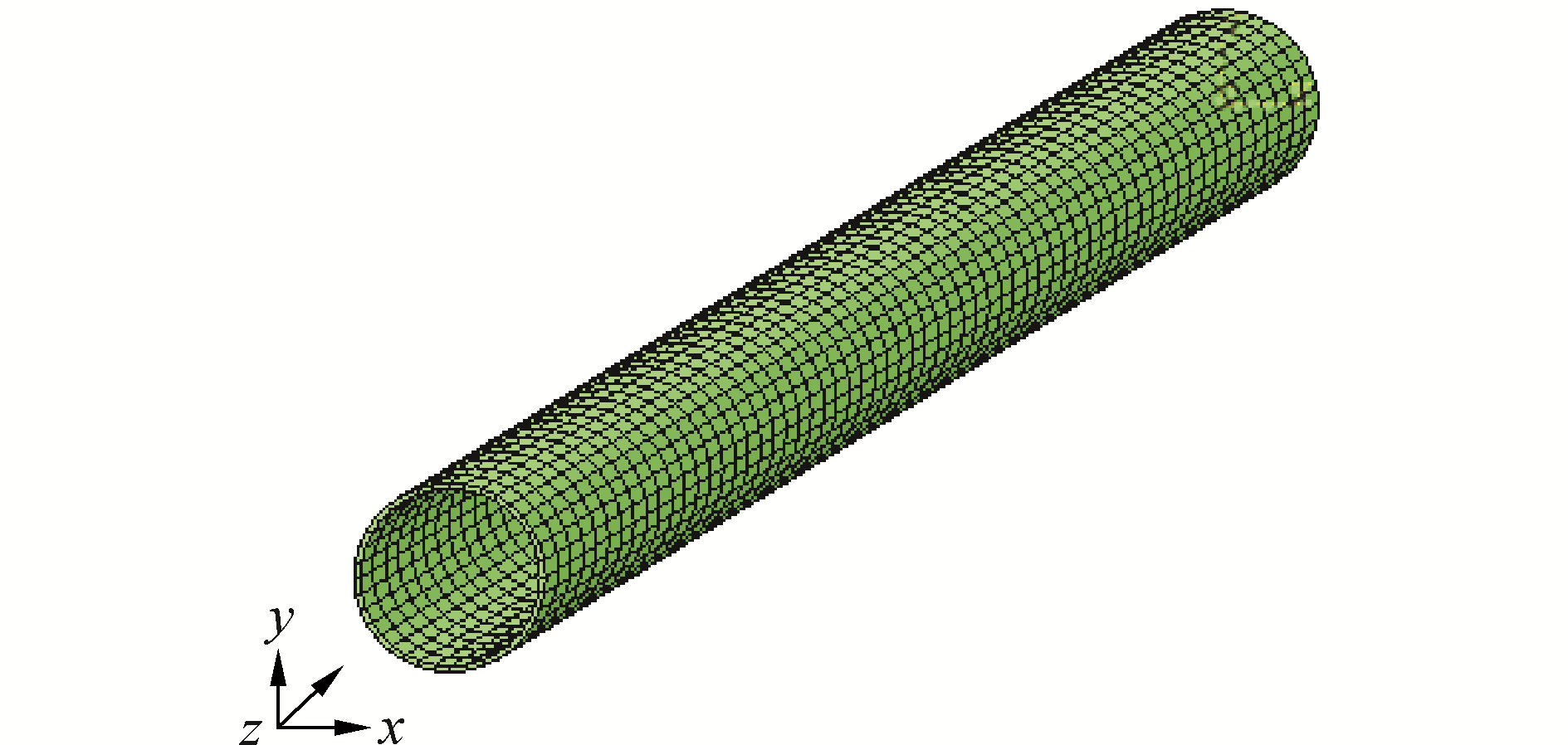Download: 图 7 PVC管道的有限元模型 Fig. 7 View of finite element model of PVC pipeline
2.2.2 验证结果分析Download: 图 8 基于模型试验与数值模拟的振型对比图 Fig. 8 Comparison of mode shapes vertically based on test and numerical simulation
3 改进模型的应用

3.1 建立损伤管道与海床相互作用的模型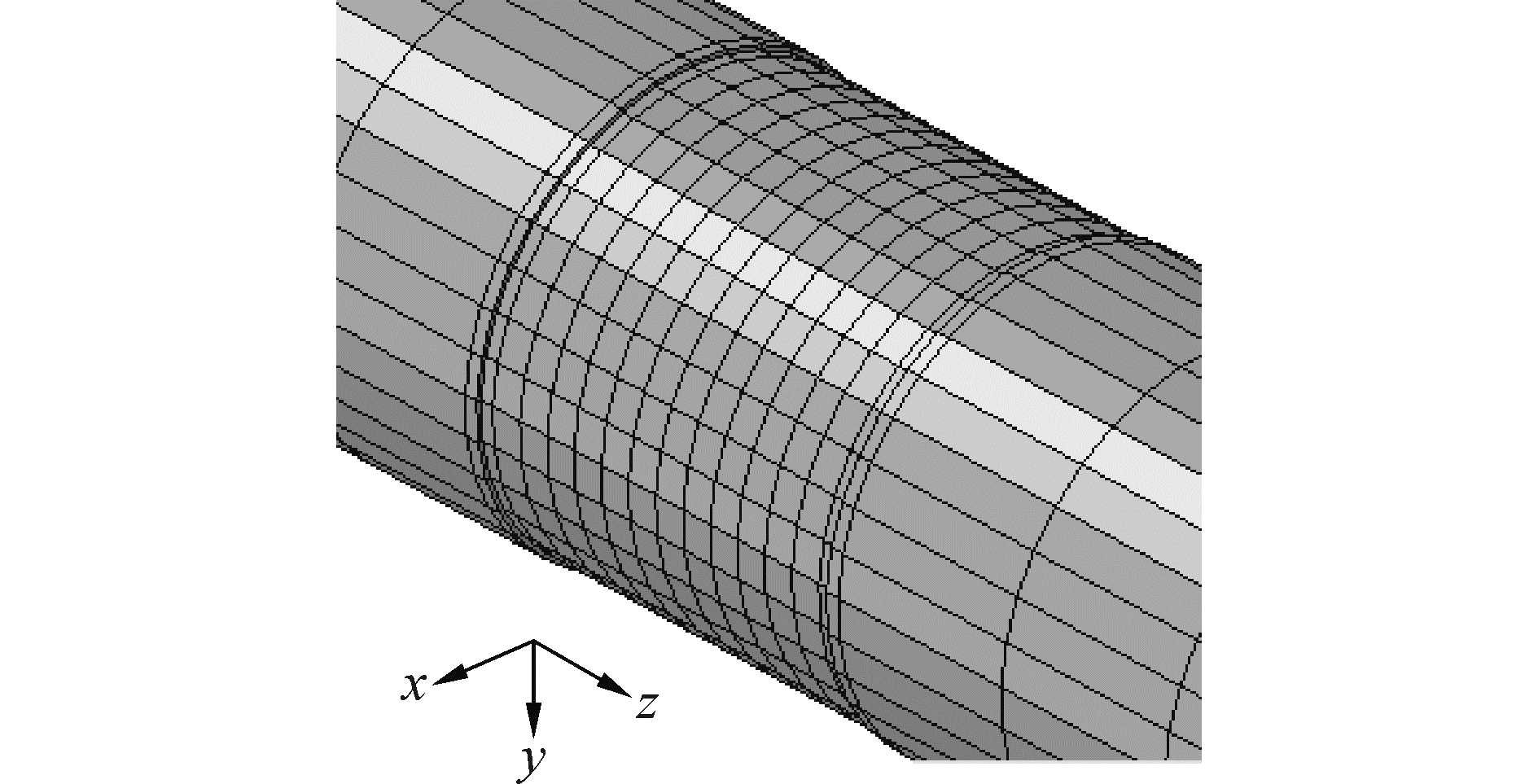Download: 图 9 海底管道体积损伤部位的完整模型 Fig. 9 Finite element model of pipeline in damaged zone

3.2 结果分析 3.2.1 位移响应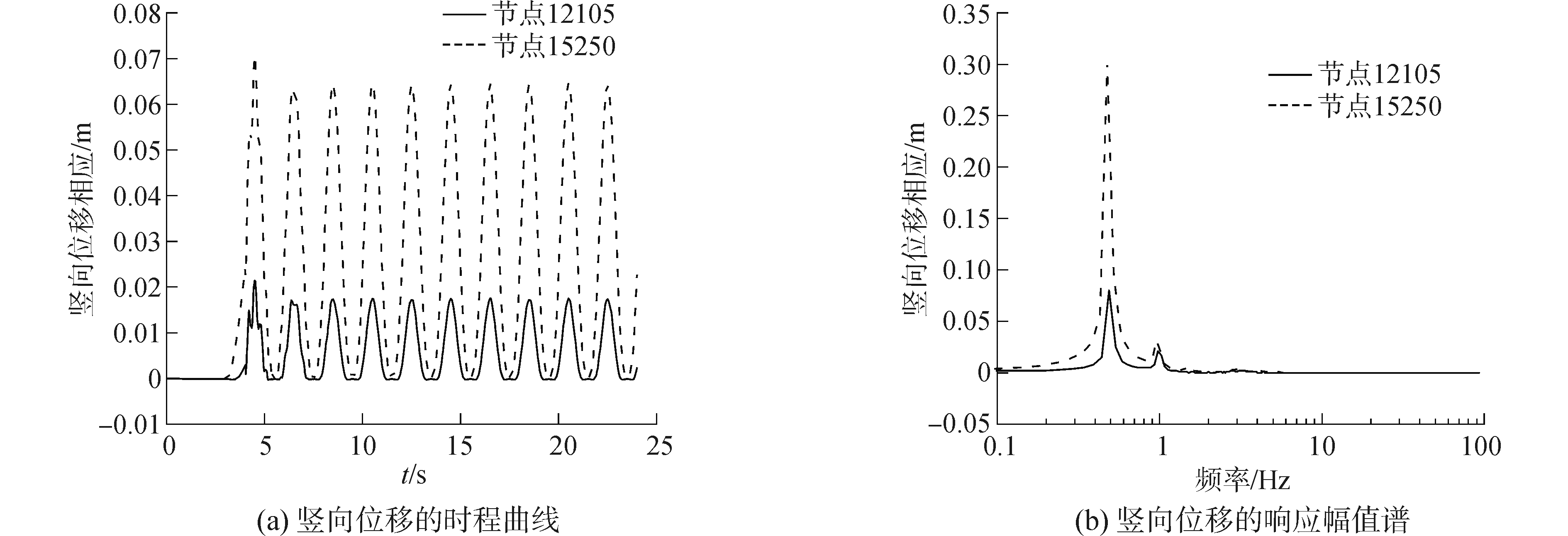Download: 图 10 节点12105和15250的位移响应 Fig. 10 Displacement response of node 12105 and node 15250
3.2.2 应力响应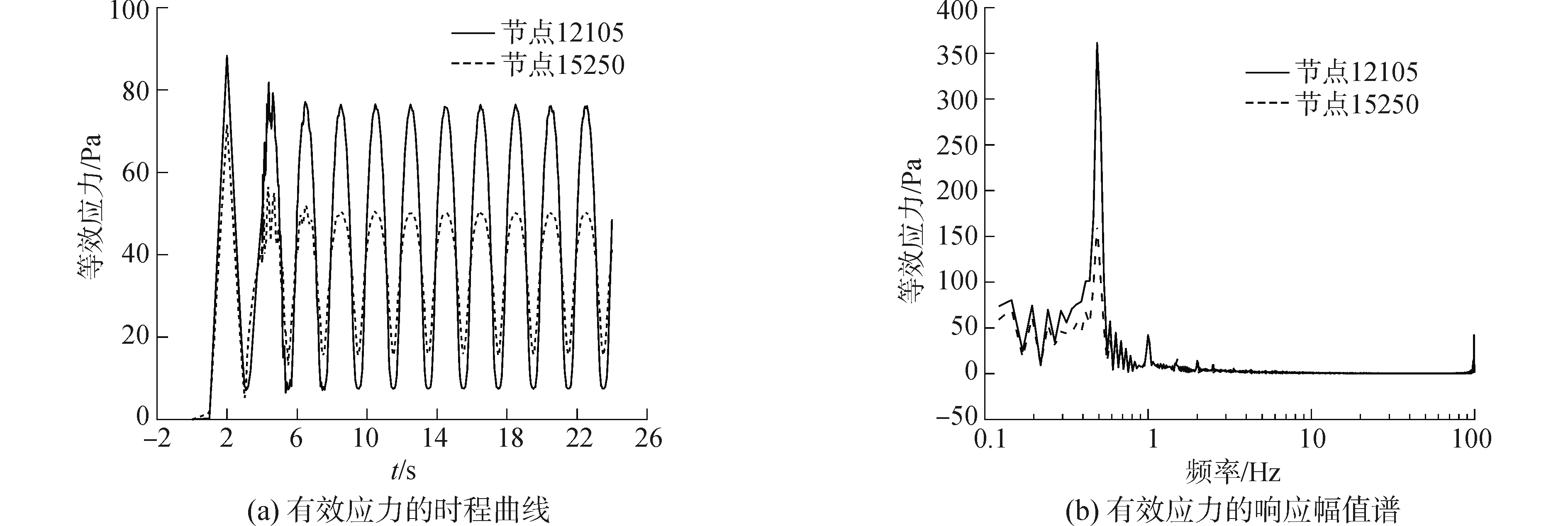Download: 图 11 节点12105和15250的有效应力响应 Fig. 11 Stress response of node 12105 and node 15250

4 结论

1) 改进模型在数值计算过程中考虑管材和海床在相互作用过程中的材料非线性、几何非线性以及接触非线性，使计算结果更加符合工程实际。

2) 改进模型充分考虑了触地段管道较大的位移载荷和弯曲变形，更加符合立管质量和海床约束力的连续分布的特点。

3) 改进模型不仅可以模拟体积缺陷等损伤管道，还可以模拟管道所受的内压、外压等载荷，进而对管道进行深度分析。

  XIA Jie, DAS P K, KARUNAKARAN D. A parametric design study for a semi/SCR system in Northern North Sea[J]. Ocean engineering, 2008, 35(17/18): 1686-1699. (0)  PALMER A. Touchdown indentation of the seabed[J]. Applied ocean research, 2008, 30(3): 235-238. DOI:10.1016/j.apor.2008.09.004 (0)  郭海燕, 高秦岭, 王小东. 钢悬链线立管与海床土体接触问题的ANSYS有限元分析[J]. 中国海洋大学学报, 2009, 39(3): 521-525. GUO Haiyan, GAO Qinling, WANG Xiaodong. Finite element analysis of steel catenary riser/soil contact problem by ANSYS[J]. Periodical of Ocean University of China, 2009, 39(3): 521-525. (0)  黄维平, 孟庆飞, 白兴兰. 钢悬链式立管与海床相互作用模拟方法研究[J]. 工程力学, 2013, 30(2): 14-18. HUANG Weiping, MENG Qingfei, BAI Xinglan. The simulation methods of the interaction of steel catenary risers and soil in touchdown down zone[J]. Engineering mechanics, 2013, 30(2): 14-18. (0)  HAWLADER B, FOUZDER A, DUTTA S. Numerical modeling of suction and trench formation at the touchdown zone of steel catenary riser[J]. International journal of geomechanics, 2016, 16(1): 04015033. DOI:10.1061/(ASCE)GM.1943-5622.0000497 (0)  杜金新, LOW Y M. 海洋立管-海床土体接触作用数值分析[J]. 工程地质计算机应用, 2008(4): 6-11. DU Jinxin, LOW Y M. Numerical analysis the interaction of risers and seabed[J]. Calculating machine application on engineering and geology, 2008(4): 6-11. (0)  PESCE C P, MARTINS C A. Riser-soil interaction: Local dynamics at TDP and a discussion on the eigenvalue and the VIV problem[C]//Proceedings of the 23rd International Conference on Offshore Mechanics and Arctic Engineering. Vancouver: ASME, 2004: 583-594. (0)  BAI Xinglan, HUANG Weiping, VAZ M A, et al. Riser-soil interaction model effects on the dynamic behavior of a steel catenary riser[J]. Marine structures, 2015, 41: 53-76. DOI:10.1016/j.marstruc.2014.12.003 (0)  白兴兰, 黄维平, 高若沉. 海床土刚度对钢悬链线立管触地点动力响应的影响分析[J]. 工程力学, 2011, 28(S1): 211-216. BAI Xinglan, HUANG Weiping, GAO Ruochen. Effect of seabed soil stiffness on dynamic response of a steel catenary riser at touchdown point[J]. Engineering mechanics, 2011, 28(S1): 211-216. (0)  余同希, 邱信明. 冲击动力学[M]. 北京: 清华大学出版社, 2011. YU Tongxi, QIU Xinming. Dynamic in shock[M]. Beijing: Tsinghua University Press, 2011. (0)  JOHNSON K L. Contact mechanics[M]. Cambridge: University Press, 1985. (0)  毛海英, 郭海燕, 赵伟. 钢悬链线立管触地点区域管土动力相互作用分析[J]. 厦门大学学报(自然科学版), 2015, 54(1): 133-137. MAO Haiying, GUO Haiyan, ZHAO Wei. Analysis of steel catenary riser/seafloor interaction in the touch down zone[J]. Journal of Xiamen University (natural science), 2015, 54(1): 133-137. (0)  郑志昌, 陈俊仁, 朱照宇. 南海海底土体物理力学特征及其地质环境初步研究[J]. 水文地质工程地质, 2004, 31(4): 50-53, 65. ZHENG Zhichang, CHEN Junren, ZHU Zhaoyu. Physical and mechanical characteristics of seabed soils and its geological environment in South China Sea[J]. Hydrogeology and engineering geology, 2004, 31(4): 50-53, 65. DOI:10.3969/j.issn.1000-3665.2004.04.008 (0)